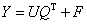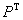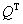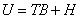Home News About Me Ph. D. Thesis Abstract Table of Contents 1. Introduction 2. Theory  Fundamentals of the Multivariate Data Analysis 2.1. Overview of the Multivariate Quantitative Data Analysis 2.2. Experimental Design 2.3. Data Preprocessing 2.4. Data Splitting and Validation 2.5. Calibration of Linear Relationships 2.5.1. PLS 2.6. Calibration of Nonlinear Relationships 2.7. Neural Networks  Universal Calibration Tools 2.8. Too Much Information Deteriorates Calibration 2.9. Measures of Error and Validation 3. Theory  Quantification of the Refrigerants R22 and R134a: Part I 4. Experiments, Setups and Data Sets 5. Results  Kinetic Measurements 6. Results  Multivariate Calibrations 7. Results  Genetic Algorithm Framework 8. Results  Growing Neural Network Framework 9. Results  All Data Sets 10. Results  Various Aspects of the Frameworks and Measurements 11. Summary and Outlook 12. References 13. Acknowledgements Publications Research Tutorials Downloads and Links Contact Search Site Map Print this Page### 2.5.1.   PLS

PLS, which was originally developed for IR- and UV-spectroscopy owes its wide application to its speed, robustness and user-friendliness. PLS performs a linear regression in a new coordinate system with a lower dimensionality than the original space of the independent variables. The new coordinates are called PLS factors or principal components (the latter is less correct but widely used analogous to the principal components for the PCA). The principal components are determined by the maximum variance of the independent variables and by a maximum correlation with the dependent variable(s). There are as many principal components as variables to predict, but for the actual model only the primary, most important principal components are used. This makes PLS robust to noise, as in theory the noise should be encapsulated in the less important principal components and the information of interest should be represented by the primary principal components. The actual regression is performed in the space spanned by the new reduced coordinate system of the orthogonal principal components. Different criteria exist for the number of principal components to be used: One of the easiest methods compares the corresponding eigenvalue of the principal component with the eigenvalues of the higher components using an F-test ,. Eastman et al.  used a crossvalidation method for the determination of the optimal number of principal components, which corresponds to the minimal predicted residual sum of squares. This criterion is more conservative in terms of the number of principal components and is widespread in literature. Recently, Martens et al. introduced the Martens' Uncertainty Test ,, which uses a jackknifing procedure with many sub-models to determine the significant variables and the optimal number of principal components, which are found in an iterative procedure with an elimination of instable variables. Compared with the crossvalidation criterion, the number of principal components is biased towards a lower number rendering this criterion very conservative for the selection of the principal components (see also section 6.1). Other methods use knowledge of the size of the measurement error for the estimation of the optimal number of principal components  or add artificial noise to the data and determine the optimal number of components by comparing with the original data in a bootstrapping procedure . In this study, the Martens' Uncertainty Test and the minimum crossvalidation error criterion are used.

In mathematical terms, PLS can be described as follows: The matrixes X and Y of the independent and dependent variables are decomposed according to(8)(9)

with E and F as residual matrices, T and U as score matrixes andandas loading matrixes. For the decomposition, either a singular value decomposition (SVD) or the non­linear iterative partial least squares (NIPALS) algorithm can be used - A linear model is assumed to relate the score matrixes T and U (with H as residual matrix and B as diagonal matrix):(10)

The PLS1 algorithm models one variable y at a time, whereas the PLS2 algorithm can model several variables in one run. In this study, the PLS1 algorithm is used, as multiple PLS1 models often perform better than a single PLS2 model ,. Further details of the algorithms can be found in ,,-.

 Page 41 © Frank Dieterle, 03.03.2019